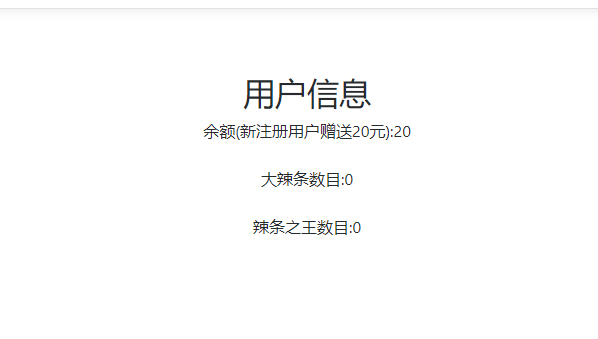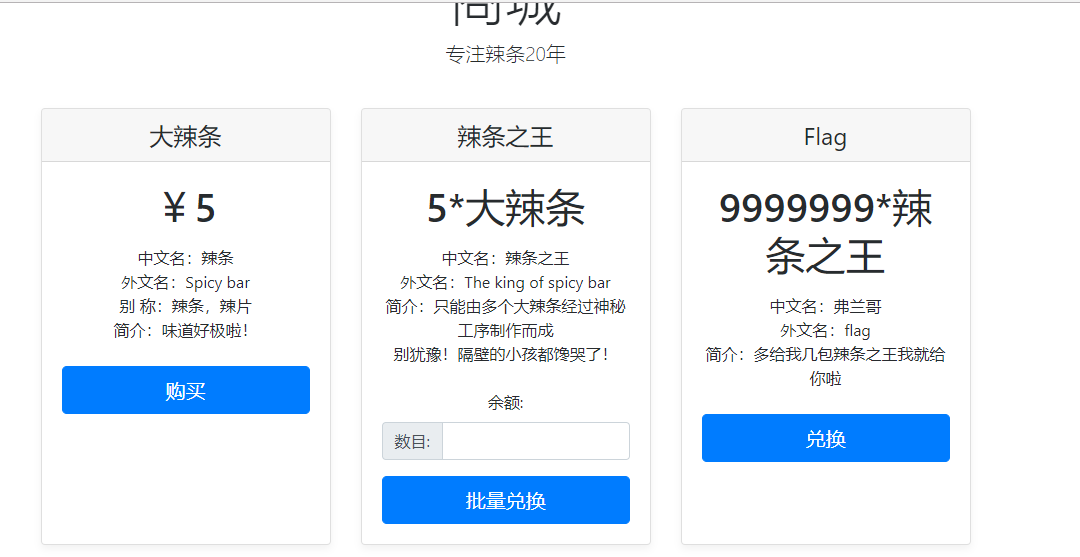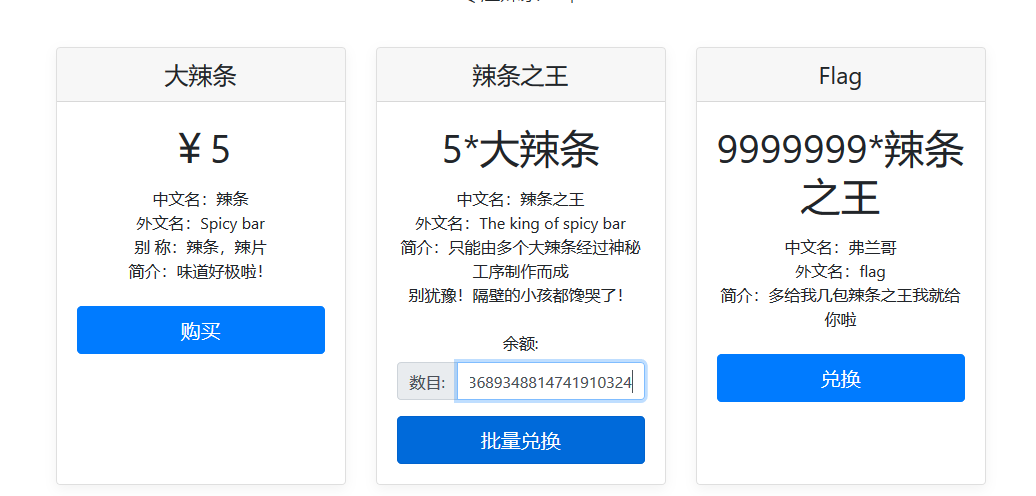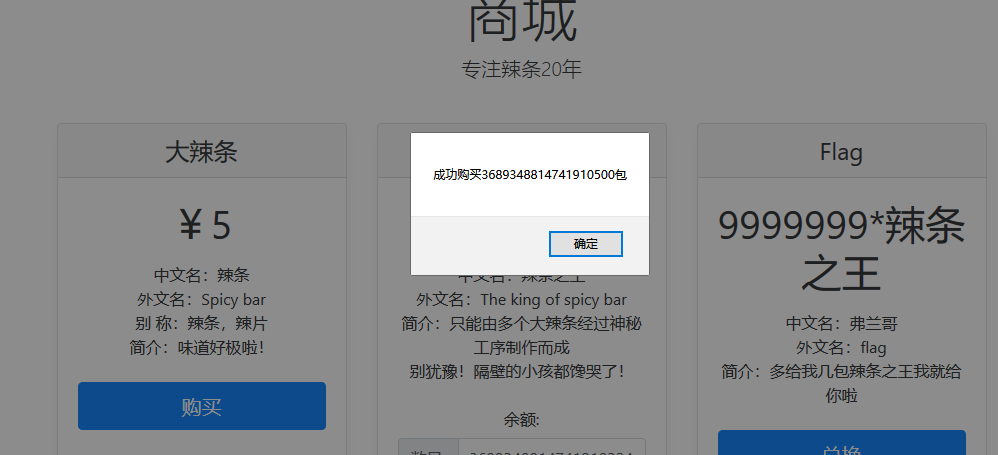# 护网杯ltshop引发的思考

### 题目描述### 做题思路

#伪代码
uint64 number = input()    //用户输入的兑换辣条之王的数字
uint64 max = math.uint64max //无符号整型的最大值
uint64 biglt = 16     //用户的大辣条包数，如上假如为16包
uint64 kinglt = 0    //用户拥有的辣条之王

if(number不是数字){
error
}
else if(number > max){
error
}
else if( (biglt - 5 * number) < 0 ){
print 买不起那么多
}
else if( (biglt - 5 * number) >= 0 ){
print 购买成功
biglt = biglt - 5 * number
kinglt = number
}

Go的64位无符号整型最大值为18446744073709551615（简称max）

• max + 1 = 0
• max + 2 = 1
• max + n = n -1
• max * 2 = max - 1
• max * 3 = max - 2
• max * n = max - n

biglt - 5 * number必须大于0